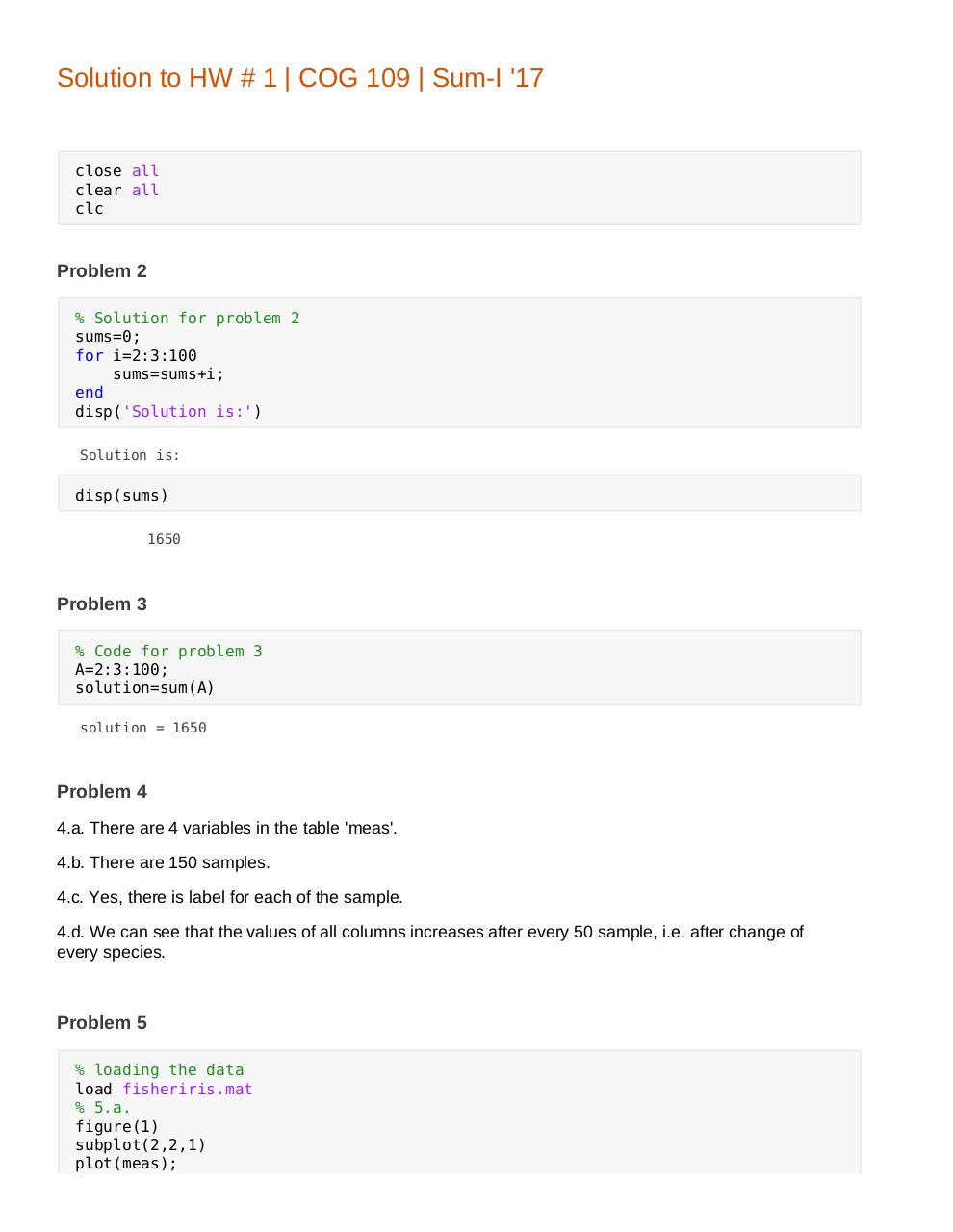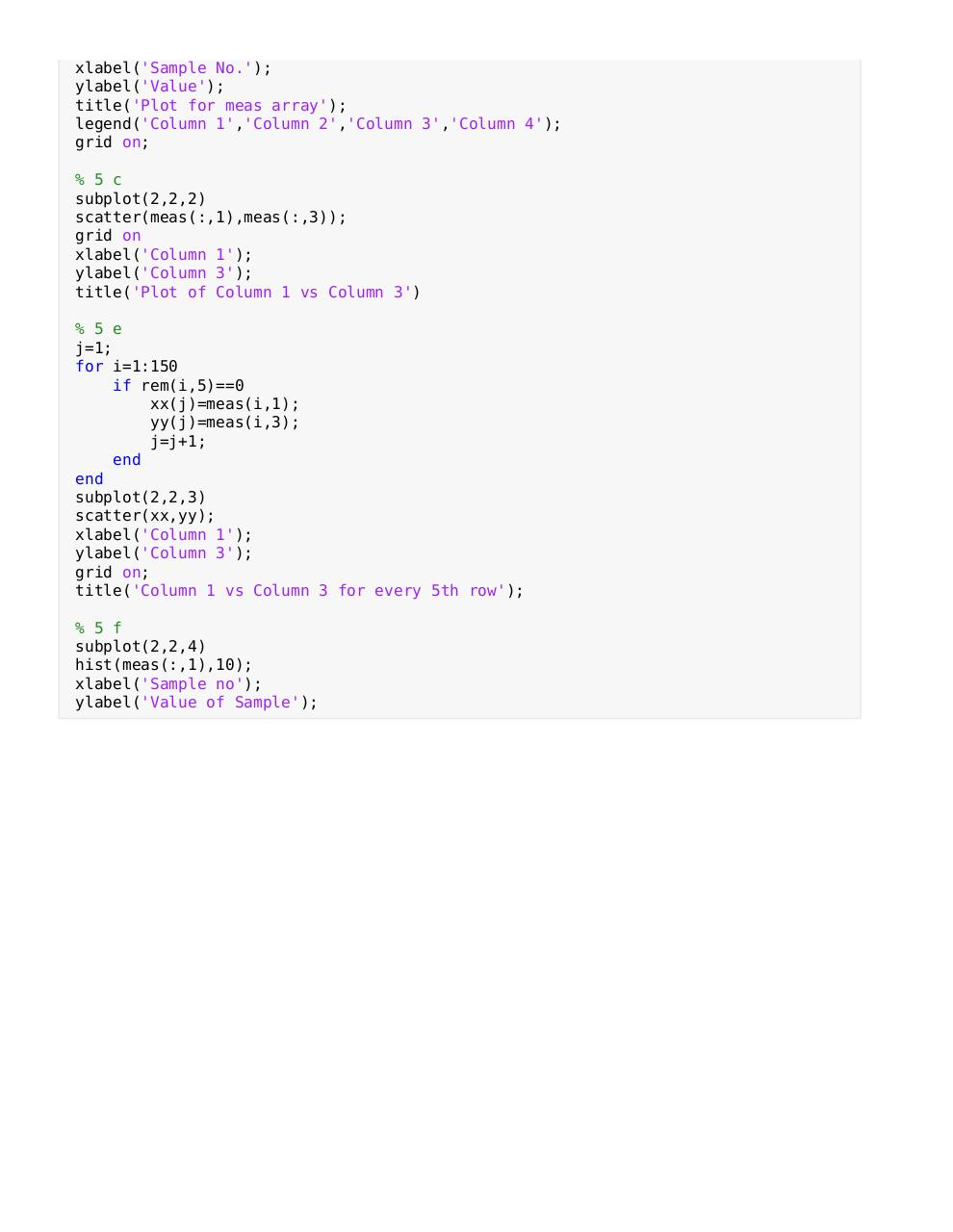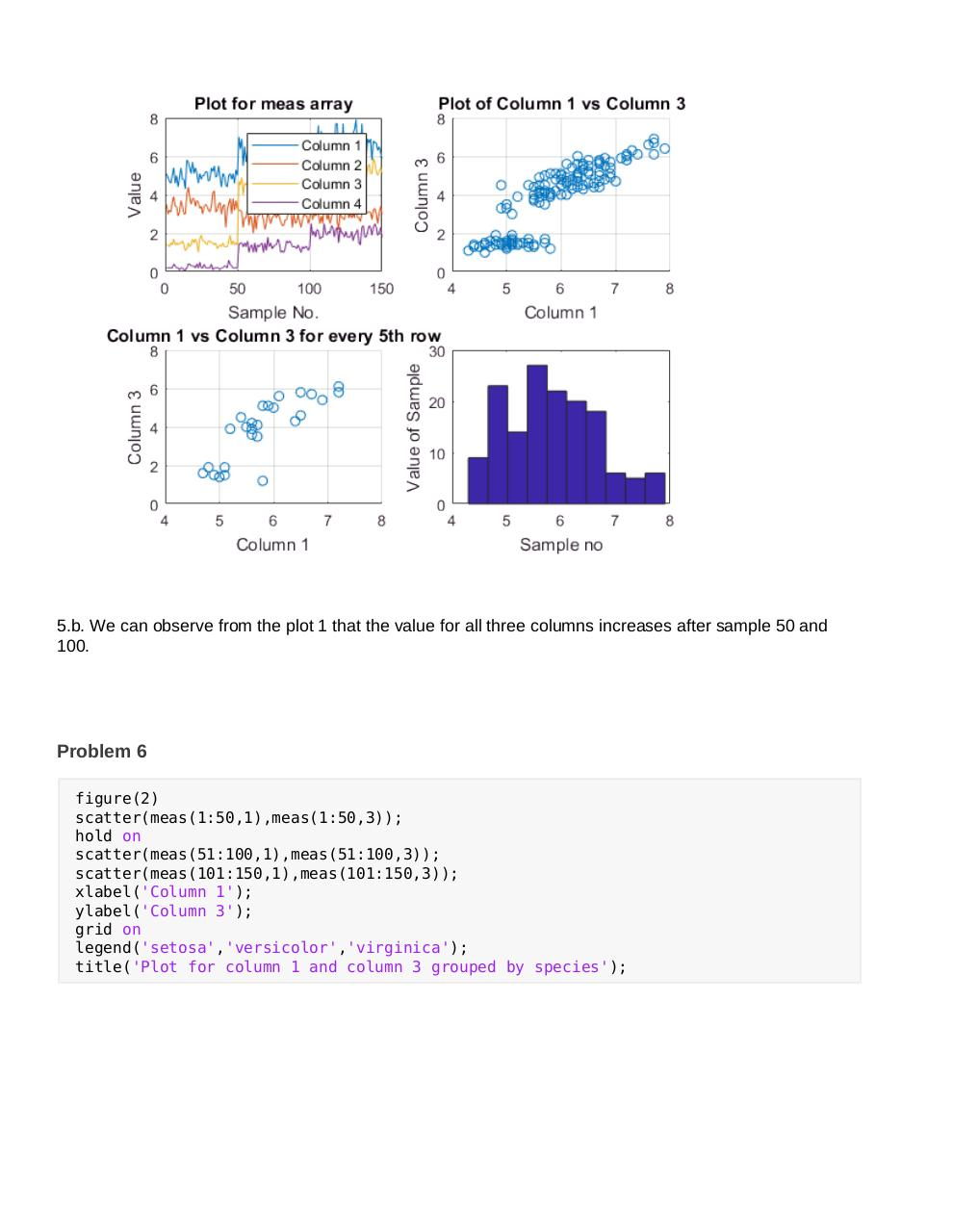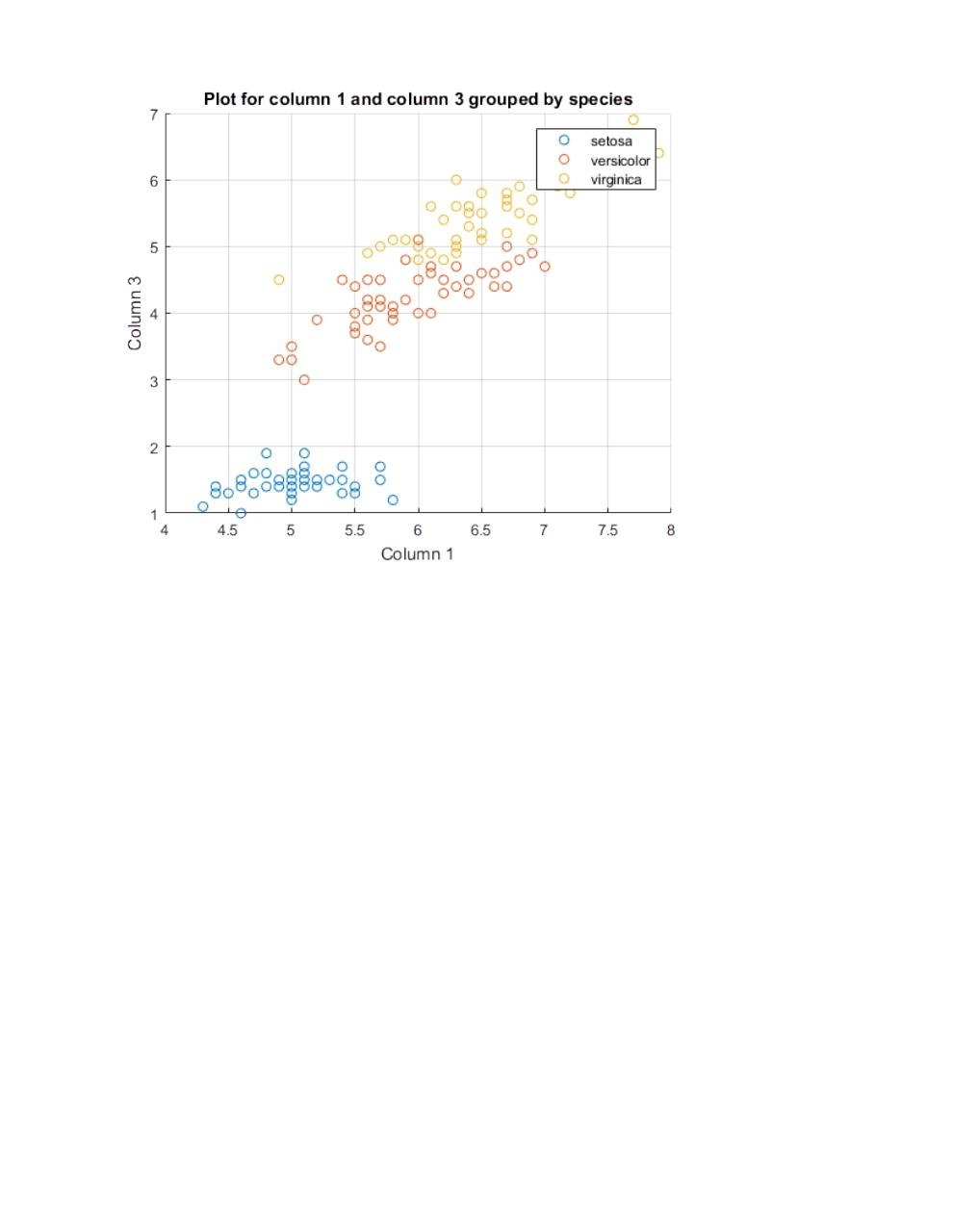# Solution HW1 .pdf

### File information

Original filename: Solution_HW1.pdf

This PDF 1.4 document has been generated by Apache FOP Version 2.2.0-SNAPSHOT, and has been sent on pdf-archive.com on 22/07/2017 at 04:23, from IP address 66.75.x.x. The current document download page has been viewed 302 times.
File size: 81 KB (4 pages).
Privacy: public file

Solution_HW1.pdf (PDF, 81 KB)

### Document preview

Solution to HW # 1 | COG 109 | Sum-I '17

close all
clear all
clc

Problem 2
% Solution for problem 2
sums=0;
for i=2:3:100
sums=sums+i;
end
disp('Solution is:')
Solution is:

disp(sums)
1650

Problem 3
% Code for problem 3
A=2:3:100;
solution=sum(A)
solution = 1650

Problem 4
4.a. There are 4 variables in the table 'meas'.
4.b. There are 150 samples.
4.c. Yes, there is label for each of the sample.
4.d. We can see that the values of all columns increases after every 50 sample, i.e. after change of
every species.

Problem 5
% 5.a.
figure(1)
subplot(2,2,1)
plot(meas);

xlabel('Sample No.');
ylabel('Value');
title('Plot for meas array');
legend('Column 1','Column 2','Column 3','Column 4');
grid on;

% 5 c
subplot(2,2,2)
scatter(meas(:,1),meas(:,3));
grid on
xlabel('Column 1');
ylabel('Column 3');
title('Plot of Column 1 vs Column 3')

% 5 e
j=1;
for i=1:150
if rem(i,5)==0
xx(j)=meas(i,1);
yy(j)=meas(i,3);
j=j+1;
end
end
subplot(2,2,3)
scatter(xx,yy);
xlabel('Column 1');
ylabel('Column 3');
grid on;
title('Column 1 vs Column 3 for every 5th row');

% 5 f
subplot(2,2,4)
hist(meas(:,1),10);
xlabel('Sample no');
ylabel('Value of Sample');

5.b. We can observe from the plot 1 that the value for all three columns increases after sample 50 and
100.

Problem 6
figure(2)
scatter(meas(1:50,1),meas(1:50,3));
hold on
scatter(meas(51:100,1),meas(51:100,3));
scatter(meas(101:150,1),meas(101:150,3));
xlabel('Column 1');
ylabel('Column 3');
grid on
legend('setosa','versicolor','virginica');
title('Plot for column 1 and column 3 grouped by species');#### HTML Code

Copy the following HTML code to share your document on a Website or Blog

#### QR Code### Related keywords